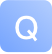2018-12-10 13:58:39 1147 1

/**

• Compute the probabilities of each value on the given [[DataSet]]
*
• @param x single colum [[DataSet]]
• @return Sequence of probabilites for each value
*/

private[this] def probs(x: DataSet[Double]): Seq[Double] = {

``````    val counts = x.groupBy(_.doubleValue)
.reduceGroup(_.size.toDouble)
.name("X Probs")
.collect

val total = counts.sum

counts.map(_ / total)``````

}

/**

• Computes 'symmetrical uncertainty' (SU) - a symmetric mutual information measure.
*
• It is defined as SU(X, y) = 2 * (IG(X|Y) / (H(X) + H(Y)))
*
• @param xy [[DataSet]] with two features
• @return SU value
*/

def symmetricalUncertainty(xy: DataSet[(Double, Double)]): Double = {

``````val su = xy.mapPartitionWith {
case in ⇒
val x = in map (_._2)
val y = in map (_._1)

val mu = mutualInformation(x, y)
val Hx = entropy(x)
val Hy = entropy(y)

Some(2 * mu / (Hx + Hy))
}

}取消 提交回答

2019-07-17 23:19:15

它使用n级并行：

def symmetricalUncertainty(xy: DataSet[(Double, Double)]): Double = {

``````val su = xy.reduceGroup { in ⇒
val invec = in.toVector
val x = invec map (_._2)
val y = invec map (_._1)

val mu = mutualInformation(x, y)
val Hx = entropy(x)
val Hy = entropy(y)

2 * mu / (Hx + Hy)
}

}
您可以在InformationTheory.scala及其测试InformationTheorySpec.scala中查看整个代码

0 0

5

Spark 【问答合集】

38

2

3

Kafka、ActiveMQ、RabbitMQ、RocketMQ的区别？【Java问答学堂】19期

1

8

2

10

【6.27更新】你不能错过的：阿里中间件性能挑战赛重要学习资料

2

56

0

377

JS零基础入门教程（上册）